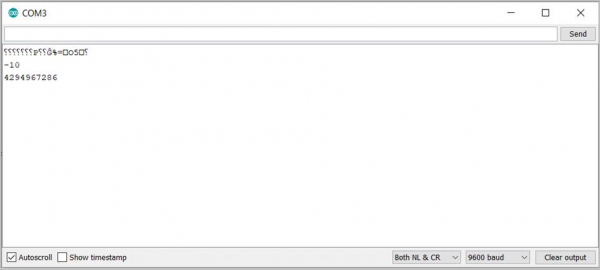# Difference between signed and unsigned integer in Arduino

When you define an integer, it is signed by default. In other words, it can accept both positive and negative values. Unsigned integers, as the name suggests, accept only positive values. Therefore, they have a higher range.

If you are using a board that uses two bytes (16 bits) to represent an integer, then the maximum range you would get for an unsigned integer is 0 to 65535 (216-1).

However, when representing signed integers, the range would be -32767 to +32767. Note that 32767 corresponds to (215 -1). As you can see, the most significant bit seems to be out of action. The most significant bit actually is used to determine the sign (0 for positive numbers and 1 for negative numbers) and the remaining 15 bits represent the value of the number, using the 2’s complement math. You can read more about it here: https://en.wikipedia.org/wiki/Two%27s_complement.

Similarly, if the board you are using represents an integer using 4 bytes (32 bits), the unsigned integers will range from 0 to 4,294,967,295 (232 - 1) and the signed integers will range from – 2147483647 to +2147483647. Note that 2147483647 corresponds to (231 - 1).

If you try to subtract a higher number from a lower number, and try to assign the result to an unsigned integer, it will result in an integer overflow (because unsigned integers cannot handle negative numbers). See the below code −

## Example

void setup() {
Serial.begin(9600);
Serial.println();
int x = 10;
int y = 20;
int z = x-y;
unsigned int w = x-y;
Serial.println(z);
Serial.println(w);
}
void loop() {
// put your main code here, to run repeatedly:
}

The Serial Monitor output is −

OutputAs you can see, the integer z was able to store a negative value. But the unsigned integer w was unable to do that, and it instead printed (232 – 10) instead of -10.